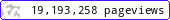•••••MadAsMaths.com :: I.Y.G.B. Practice Papers :: A Level Practice Papers 2019 Spec :: FS1 Practice Papers

I.Y.G.B. FS1 PRACTICE PAPERS

These I.Y.G.B. practice papers mostly follow the Pearson/Edexcel Syllabus introduced for teaching from 2017.

Some modules will be first examined in 2018 and some in 2019.

• Papers A to R have standard difficulty with later papers usually more difficult.
• Papers S and T are extremely hard.
• Papers U to Z are hard.

All papers have model solutions.

 fs1_a.pdffs1_a_solutions.pdffs1_b.pdffs1_b_solutions.pdffs1_c.pdffs1_c_solutions.pdffs1_d.pdffs1_d_solutions.pdffs1_e.pdffs1_e_solutions.pdffs1_f.pdffs1_f_solutions.pdffs1_g.pdffs1_g_solutions.pdffs1_h.pdffs1_h_solutions.pdffs1_i.pdffs1_i_solutions.pdffs1_j.pdffs1_j_solutions.pdffs1_k.pdffs1_k_solutions.pdffs1_l.pdffs1_l_solutions.pdffs1_m.pdffs1_m_solutions.pdffs1_n.pdffs1_n_solutions.pdffs1_o.pdffs1_o_solutions.pdffs1_p.pdffs1_p_solutions.pdffs1_q.pdffs1_q_solutions.pdffs1_r.pdffs1_r_solutions.pdffs1_s.pdffs1_s_solutions.pdffs1_t.pdffs1_t_solutions.pdffs1_u.pdffs1_u_solutions.pdffs1_v.pdffs1_v_solutions.pdffs1_w.pdffs1_w_solutions.pdffs1_x.pdffs1_x_solutions.pdffs1_y.pdffs1_y_solutions.pdffs1_z.pdffs1_z_solutions.pdf